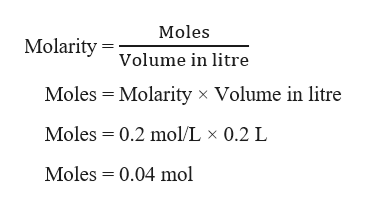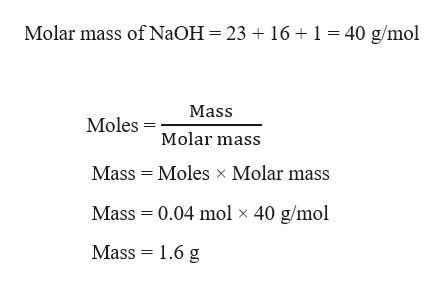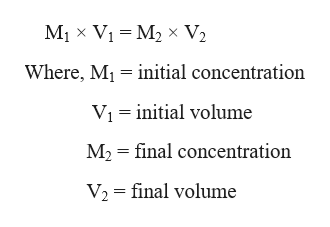# Please calculate the amount of solid sodium hydroxide needed to make 200mL of a 0.2 M soulution. **Please give answer to nearest .001 g**Also, if 10 mL of the solution made in the last question is used to make another 125mL solution of NaOH, what is the new concentration?If you dont mind, please show the each step to solve the problem, that would be great! Have a absolutely amazing day whoever you are that does these questions for students like me. :)

Question
26 views

Please calculate the amount of solid sodium hydroxide needed to make 200mL of a 0.2 M soulution. **Please give answer to nearest .001 g**

Also, if 10 mL of the solution made in the last question is used to make another 125mL solution of NaOH, what is the new concentration?

If you dont mind, please show the each step to solve the problem, that would be great!

Have a absolutely amazing day whoever you are that does these questions for students like me. :)

check_circle

star
star
star
star
star
1 Rating
Step 1

Given,

Volume of NaOH = 200 mL = 0.200 L       (1 mL = 0.001 L)

Molarity of NaOH = 0.2 M = 0.2 mol/L      (1 M = 1 mol/L)

Firstly, calculating the moles of NaOH required using the molarity formula as:help_outlineImage TranscriptioncloseMoles MolarityVolume in litre Moles Molarity x Volume in litre Moles 0.2 mol/L x 0.2 L Moles 0.04 mol fullscreen
Step 2

The amount of NaOH required in grams can be calculated as:help_outlineImage TranscriptioncloseMolar mass of N2OH = 23 + 16 1 = 40 g/mol Mass MolesMolar mass Mass Moles x Molar mass Mass 0.04 mol x 40 g/mol Mass 1.6 g fullscreen
Step 3

Now, if 10 mL of the solution made in the last question is used to make another 125mL solution of NaOH, which means that 10 mL o...help_outlineImage TranscriptioncloseM1 x Vi M2 x V2 Where, Mi initial concentration Vi initial volume M2 final concentration V2 final volume fullscreen

### Want to see the full answer?

See Solution

#### Want to see this answer and more?

Solutions are written by subject experts who are available 24/7. Questions are typically answered within 1 hour.*

See Solution
*Response times may vary by subject and question.
Tagged in

### Solutions